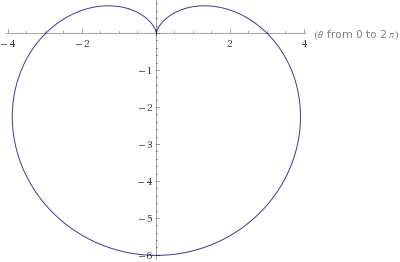# Section 4.3 Calculus 2

Polar Coordinates

## 4.3 Polar Coordinates

### 4.3.1 Definition of Polar Coordinates

• The polar coordinate $$p(r,\theta)$$ is defined to be Cartesian coordinate $$(r\cos\theta,r\sin\theta)$$.
• Example Plot the polar coordinates $$p(2,\pi/3),p(\sqrt{2},3\pi/4),p(-2,4\pi/3),p(4,11\pi/6)$$.
• Note that every polar coordinate $$p(r,\theta)$$ is equal to $$p(r,\theta+k\pi)$$ for all even integers $$k$$, and equal to $$p(-r,\theta+k\pi)$$ for all odd integers $$k$$.

### 4.3.2 Equations Relating Polar and Cartesian Coordinates

• Polar and Cartesian coordinates may be related by the equations $$x=r\cos\theta$$, $$y=r\sin\theta$$, $$x^2+y^2=r^2$$, and $$\tan\theta=\frac{y}{x}$$.
• Example Convert the Cartesian coordinate $$(-2\sqrt3,2)$$ into a polar coordinate.
• Example Convert the polar equation $$r=\frac{1}{\sin\theta-\cos\theta}$$, $$\pi/4<\theta<5\pi/4$$ into a Cartesian equation.
• Example Convert the Cartesian equation $$(x-2)^2+y^2=4$$ into a polar equation.

### 4.3.3 Common Polar Equations

• The equation $$r=R$$ is a circle centered at the origin of radius $$R$$.
• The equation $$r=D\cos\theta$$ is the circle with diameter $$D$$ tangent to the $$y$$-axis at the origin.
• The equation $$r=D\sin\theta$$ is the circle with diameter $$D$$ tangent to the $$x$$-axis at the origin.
• The equation $$\theta=\alpha$$ is the line passing through the origin at the angle $$\alpha$$.
• The equation $$r\cos\theta=a$$ is the vertical line $$x=a$$.
• The equation $$r\sin\theta=a$$ is the horizontal line $$y=a$$.
• Example Sketch the region where $$0<\csc\theta\leq r\leq 2$$.
• The equations $$r=a\pm a\cos\theta$$ and $$r=a\pm a\sin\theta$$ are known as cardioids.
• Example Sketch the cardioid $$r=4-4\sin\theta$$.

### Review Exercises

1. Convert the polar coordinates $$p(\sqrt 3,2\pi/3),p(\sqrt 2,\pi/4),p(2,7\pi/6),p(-\sqrt 3,-\pi/3)$$ to Cartesian and plot them in the $$xy$$ plane.
2. Convert the Cartesian coordinates $$(4,-4),(-\frac{3}{2},-\frac{\sqrt 3}{2})$$ into polar coordinates.
3. Convert the polar equation $$r=\frac{5}{\sqrt{25-9\sin^2\theta}}$$ into a Cartesian equation. Name the curve.
4. Convert the Cartesian equation $$1-\frac{y}{x^2+y^2}=\frac{3}{\sqrt{x^2+y^2}}$$ into a polar equation.
5. Convert the Cartesian equation for the line $$y=\frac{x}{\sqrt 3}$$ into a polar equation.
6. Sketch the region where $$0< 3\sec\theta\leq r\leq 6\cos\theta$$. (Hint: Completing the square in $$x^2-6x+y^2=0$$ yields $$(x-3)^2+y^2=9$$.)
7. Sketch the cardioid $$r=3+3\sin\theta$$.
8. Sketch the cardioids $$r=1+\cos\theta$$ and $$r=1-\cos\theta$$. At what points do they intersect?
9. Sketch the “three-leaved rose” $$r=\sin 3\theta$$.
10. What polar coordinate gives the Cartesian coordinate $$(-\sqrt3,1)$$?
11. Convert the circle $$x^2+(y-4)^2=16$$ into a polar equation.
12. What equation gives the curve drawn below?Solutions 1-5

Solutions 6-12

#### Textbook References

• University Calculus: Early Transcendentals (3rd Ed)
• 10.3